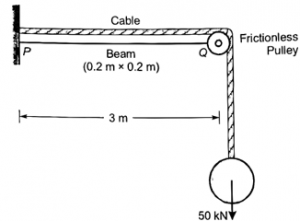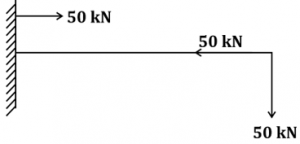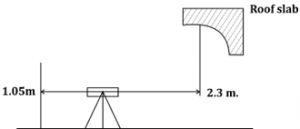Engineering Jobs   »   Free Gate Civil engineering quiz

# GATE’22 CE:- Weekly Practice Quiz 08-Aug-2021

Quiz: Civil Engineering
Exam: GATE
Topic: Miscellaneous

Each question carries 2 marks
Negative marking: 1/3 mark
Time: 20 Minutes

Q1. The values of axial stress (σ) in kN/m², bending moment (M) in kN-m, and shear force (V) in kN acting at point P for the arrangement shown in the figure are respectively(a) 1000, 75 and 25
(b) 1250, 150 and 50
(c) 1500, 225 and 75
(d) 1750, 300 and 100

Q2. At the limit state of collapse, an RC beam is subjected to flexural moment 200 kN-m, shear force 20 kN and torque 9 kN-m. the beam is 300 mm wide and has a gross depth of 425 mm, with an effective cover of 25 mm. the equivalent nominal shear stress (τ_ve) as calculated by using the design code turns out to be lesser than the design shear strength (τ_c ) of the concrete. The equivalent shear force (V_C ) is
(a) 20 kN
(b) 54 kN
(c) 56 kN
(d) 68 kN

Q3. Two equal angles ISA 100 mm × 100 mm of thickness 10 mm are placed back – to – back and connected to the either side of a gusset plate through a single row of 16 mm diameter rivets in double shear. The effective areas of the connected and unconnected legs of each of these angles are 775 mm² and 950 mm², respectively. If these angles are NOT tack riveted, the net effective area of this pair of angles is
(a) 3650 mm²
(b) 3450 mm²
(c) 3076 mm²
(d) 2899 mm²

Q4. A soil mass has coefficients of horizontal and vertical permeability as 9 × 10^(-7) cm/s and 4 × 10^(-7) cm/s respectively. The transformed coefficient of permeability of an equivalent isotropic soil mass is
(a) 4 × 10^(-7) cm/s
(b) 6 × 10^(-7) cm/s
(c) 9 × 10^(-7) cm/s
(d) 13 × 10^(-7) cm/s

Q5. If duty (D) is 1428 hectares/cumec and base period (B) is 120 days for an irrigated crop, then delta (∆) in meters is given by
(a) 0.01
(b) 1.38
(c) 0.73
(d) 102.8

Q6. The radius of relative stiffness for a 20 cm. thick slab with E = 3 ×10^5 kg/cm² and poison’s ratio = 0.15, resting on a subgrade having modulus of 5 kg/cm³ is
(a) 320 cm
(b) 120 cm
(c) 80 cm
(d) 10 cm

Q7. Isopleths are lines on a map through points having equal depth of
(a) Total runoff
(b) Evapotranspiration
(c) Rain fall
(d) Infiltration

Q8. A steady incompressible, two-dimensional velocity field is given by. U = x + y +1; v = x – y -2. The ratio of the co-ordinates (x, y) at the stagnation point is
(a) 0.33
(b) -0.33
(c) 0.5
(d) -0.5

Q9. To find the RL of a roof slab of building, staff reading was taken from a particular set-up of the levelling instrument. The readings were 1.050 m with staff on the Bench Mark and 2.300 m with staff below the roof slab and held inverted. Taking the RL of the Bench Mark as 135.15 m, the RL of the roof slab will be
(a) 129.800
(b) 131.900
(c) 134.400
(d) 138.500

Q10. The normal flows on two approach roads at an intersection are respectively 500 pcu/h and 300 pcu/h. the corresponding saturation flow is 1600 pcu/h on each road. The total lost time per single cycle is 16s. the optimum cycle time by Webster’s method is
(a) 72.5 s
(b) 58.0 s
(c) 48.0 s
(d) 19.3 s

Solutions

S1. Ans.(b)
Sol. Free body diagram of given fig. →Shear force (V) = 50 kN
Axial stress (σ) = 50/(0.2×0.2)=1250 kN\/m^2
Bending moment (M) = 50 × 3 = 150 kN-m

S2. Ans.(d)
Sol. Given,
V = 20 kN.
T = 9 kN-m
B = 300 mm = 0.3m
V_c = ?
Equivalent shear force (V_C )= V + 1.6 T/B
=20+(1.6×9)/0.3
=68 kN.

S3. Ans.(d)
Sol. When angles are not tack riveted, they will be considered as single angles connected on one side of gusset plate, then net effective area (A_e) ⇒
A_e = (A_1+kA_2 )×2
k=(3A_1)/(3A_1+A_2 ) [Given,■(A_1=775 mm^2@A_2=950 mm^2 )]
=(3×775)/((3×775+950) )
▭(k=0.71)
A_e=(775+0.71×950)×2
▭(A_e=2899 mm^2 )

S4. Ans.(b)
Sol. Co-efficient of horizontal permeability (k_H) =9×10^(-7) cm\/s
Co-efficient of vertical permeability (k_v) =4×10^(-7) cm\/s
k_XY=√(k_H.k_v )
= √(9×10^(-7)×4×10^(-7) )
▭(k_XY=6×10^(-7) cm\/s)

S5. Ans.(c)
Sol. Given,
D=1428 hec.\/cumec
B=120 days
∆ = ?
∆ =(8.64 B)/D
=(8.64×120)/1428
=0.726 m ≈0.73 m.

S6. Ans.(c)
Sol. Given,
h=20 cm.
E=3×10^5 kg\/cm^2
k=5 kg\/cm^3
μ=0.15
Now, radius of relative stiffness (l)
l= [(E h^3)/(12 k (1-μ)^2 )]^(1\/4)
=[(3×10^5×(20)^3)/(12×5(1-{0.15}^2 ) )]^(1\/4)
=79.98 cm≈80 cm.

S7. Ans.(b)
Sol. Isopleths are the line on a map through places having equal depth of “Evapotranspiration’.

S8. Ans.(b)
Sol. For stagnation point
|V ⃗ |=0;means u = 0 & v = 0
Now,
u = x + y + 1 = 0 —-(1)
v = x – y – 2 = 0 —–(2)
By solving equation (1) & (2), we get
x =1/2& y= (-3)/2
Now,
x/y=(1/2)/((-3)/2)=(-1)/3= -0.33

S9. Ans.(d)
Sol.RL of Benchmark = 135.15 m
BS = 1.05 m
FS = -2.30 m (Inverted)
Height of instrument (H.I) = RL + BS
= 135.15 + 1.05
= 136.20 m.
Reduce level of roof slab (RL) = HI – FS
= 136.2 – (-2.3)
= 138.500 m

S10. Ans.(b)
Sol. q_1=500 pcu\/h , S_1 = 1600 pcu/h
q_2 = 300 pcu/h, 〖 S〗_2 = 1600 pcu/h
L = Total lost time = 16 sec.
Optimum cycle time by webster’s method→
C=(1.5L+5)/(1-Σ q/s )
=((1.5×16)+5)/(1-[500/1600+300/1600]
▭(C=58sec.)

Sharing is caring!